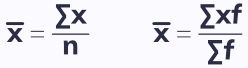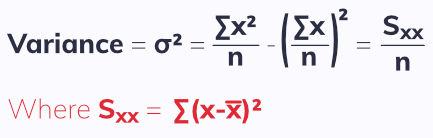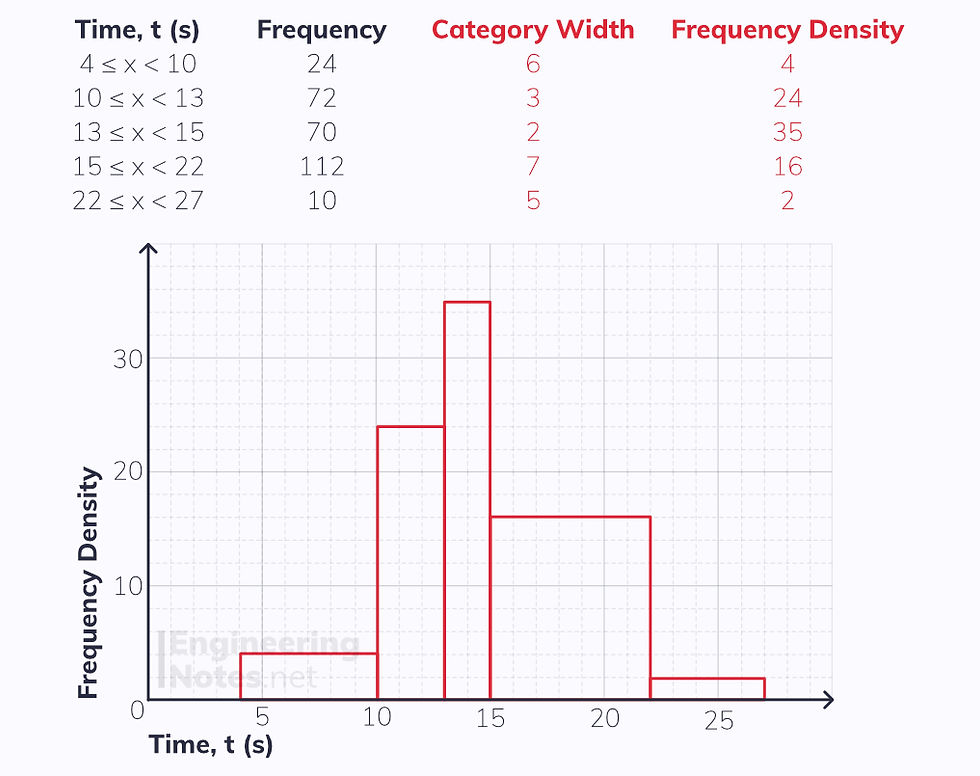top of page

#### Notes by Category University Engineering

Electronics*
Mathematics*
Mechanics & Stress Analysis*
Rate these notesNot a fanNot so goodGoodVery goodBrillRate these notes

To start off with, there is some terminology you need to be familiar with.

• A population is the whole set of items that are of interest

• A sampling unit is an individual unit of a population

• A sampling frame is a named or numbered list of the sampling units in the population.

• A quantitative variable is one associated with numerical observations

• A qualitative variable is one that is non-numerical

• A continuous variable can take any value, e.g. decimals

• A discrete variable can only take fixed values, e.g. integers or colours

• A census measures every member of a population

• A sample is a selection of observations from a subset of the population

• A census is entirely accurate (because it measures every sampling unit), but it is time consuming, cannot work with destructive testing (when the sample is destroyed when testing), and produces a vast amount of data to be processed.

• A Sample is less time consuming because less has to be tested and less data is produced, however it may not be as accurate, and the sample may not reflect the population well.

## Sampling

Broadly speaking, there are two types of sampling - random, and non-random. These each have their own sub types, too.

### Random Sampling

In random sampling, each member of a population has an equal chance of being chosen. This means the sample should be both representative and unbiased.

Simple Random Sampling

For a simple random sample, a sampling frame is created where each member is given a number. Then, a random number generator or a lottery is used to create the sample.

• Advantages are that it is free from bias, easy and cheap to use on small populations and samples, and the probability of being selected is known.

• Disadvantages are that a sampling frame needs to be constructed, and it is difficult when the population/sample is large.

Systematic Sampling

For a systematic sample, the required elements are selected at regular, chosen intervals from an ordered list. For example, if you had a population of 50 and wanted a sample of 10, use a random number generator to pick a number between one and five to find the first person, then chose every fifth after the first.

• Advantages include that it is simple and quick and works for large samples and populations

• Disadvantages include that a sampling frame is needed and, if this is not random, bias can be introduced.

Stratified Sampling

For stratified sampling, the population is divided into mutually exclusive strata, and a random sample is taken from each. These strata could be gender, eye colour etc. It is important that the proportion of each strata should be representative of the population, for example if 40% of a population are males and 60% female, a sample of 10 should have 4 males and 6 females.

• Advantages are that it reflects the population structure and gives proportional representation

• Disadvantages are that the population must be divided into mutually exclusive strata, and that the selection of members for each strata has the same issues as simple random sampling

### Non-Random Sampling

There are two main types of non-random sampling:

Quota Sampling

Quota sampling is when a researcher selects a sample that reflects the characteristics of the whole population. Individuals are screened to see which quota they fit into, and this continues until each quota is filled.

• Advantages include that it allows a small sample to represent a large population, no sampling frame is needed, it is quick and easy and allows for comparison between different groups.

• Disadvantages include that it can introduce bias, group divisions can be vastly inaccurate, and people who do not easily fit into a group are ignored.

Opportunity Sampling

Opportunity sampling, also known as convenience sampling, involves taking the sample from whoever is readily available at the time and fits the criteria. For example, this might just be the first 10 people you find.

• Advantages are that it is extremely quick and easy

• Disadvantages are that it is very unlikely to represent the population and is highly dependent on the individual researcher.

The position of something in a data set can be described using a measure of location, such as the mean, median and mode:

• The mode is the value that occurs most often

• The median is the middle value when data points are in order

• The mean is calculated using:### Variance & Standard Deviation

The variance is a measure used to describe the spread of a data set:The standard deviation is the square root of the variance:• Generally, it is easiest to use the first form of the equation (without the Sxx) when you have raw data.

• The second one (with Sxx) is best used when you can use a calculator to find out Sxx quickly.

When working with frequencies, use this equation for the variance, σ², instead:Again, standard deviation, σ, is given as the square root of this.

### Ranges

Another form of describing the spread of a data set is using ranges.

• 'The' range is the difference between the largest and the smallest value

• Interquartile range is the difference between the upper and lower quartile, Q₃ - Q₁

• Interpercentile range is the difference between the values at two given percentages

Range is a good measure because it takes into account all the data, but it can be very unreliable at times, as it is affected considerably by extreme values (outliers). Interquartile range is therefore better, as it ignores extreme values and only looks at the central 50% of data. Often, the 10th to 90th percentile range is used as it also ignored outliers, but covers 80% of data rather than 50%.

You can estimate percentiles and ranges by interpolation. This assumes the data is evenly distributed.

When working with quartiles and percentiles, if the value you calculate for the quartile/percentile is a whole number, add a half to it. If it is a decimal, round up.

### Coding

Statistical calculations can be simplified by coding each data value to make a new data set that is easier to work with.• Where a and b are constants

## Box Plots

An outlier is an extreme data point that does not match the trend of the other result. Generally, a value is defined as an outlier if it is some multiple, k, of the interquartile range (IQR) above or below the upper and lower quartiles respectively:

A value is an outlier if it is > Q₃ + k(Q₃-Q₁) or < Q₁ - k(Q₃-Q₁)

A box plot is a visual representation of a data set, and shows all the key measures clearly:Box plots are great ways of comparing different data sets:The diagram clearly shows key features for comparison:

• The two data sets share the same median

• The red set has a larger IQR

• The red set has fewer outliers

## Cumulative Frequency

When the data you are given is grouped into frequencies, you need to draw a cumulative frequency diagram to estimate the median and quartiles.

Always plot the upper class boundary on cumulative frequency diagrams## Histograms

Histograms are used to represent grouped continuous data. They are good as visual representations for data, because they show clearly where and how it is distributed.

Area ∝ frequency
Frequency density = frequency / category widthJoining the top middle of each bar with a straight line gives the frequency polygon.See All